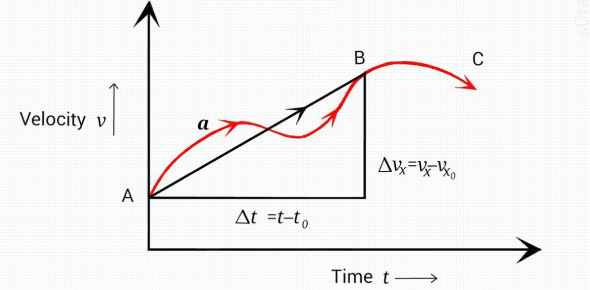# Equations Of Motion Quiz: Test!

12 Questions | Total Attempts: 462Settings.

Questions and Answers
• 1.
The diagram shows a speed-time graph for a body moving with constant acceleration.
• A.

Acceleration

• B.

Distance

• C.

Speed

• D.

Time

• 2.
A tunnel has a length of 50 km. A car takes 20 min to travel between the two ends of the tunnel. What is the average speed of the car?
• A.

2.5 km/h

• B.

16.6 km/h

• C.

150 km/h

• D.

1000 km/h

• 3.
A small steel ball is dropped from a low balcony. Ignoring air resistance, which statement describes its motion?
• A.

It falls with constant acceleration.

• B.

It falls with constant speed.

• C.

It falls with decreasing acceleration.

• D.

It falls with decreasing speed.

• 4.
The diagram shows the speed-time graph for an object moving at constant speed.What is the distance in meters travelled by the object in the first 3s?
• 5.
A car accelerates from traffic lights. The graph shows how the car’s speed changes with time.How far does the car travel before it reaches a steady speed?
• 6.
The graph represents the movement of a body accelerating from rest.After 5 seconds how far has the body moved?
• 7.
A child is standing on the platform of a station, watching the trains.A train travelling at 30m/s takes 3s to pass the child.What is the length of the train?
• 8.
The graph shows the progress of an athlete in a 100m race.What time was taken to travel 10m from the start?
• A.

2.4 s

• B.

2.8 s

• C.

65 s

• D.

70 s

• 9.
The speed-time graph shown is for a bus travelling between stops. Where on the graph is the acceleration of the bus the greatest?
• A.

A

• B.

B

• C.

C

• D.

D

• 10.
The circuit of a motor racing track is 3 km in length. In a race, a car goes 25 times round the circuit in 30 minutes. What is the average speed of the car?
• A.

75 km/h

• B.

90 km/h

• C.

150 km/h

• D.

750 km/h

• 11.
An object moves initially with constant speed and then with constant acceleration. Which graph shows this motion?
• A.

A

• B.

B

• C.

C

• D.

D

• 12.
. A car is moving downhill along a road at a constant speed. Which graph is the speed/ time graph for the car?
• A.

A

• B.

B

• C.

C

• D.

D

Related Topics